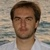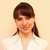# Posts tagged "Average"## Excel AVERAGEIFS function with multiple criteria

When it comes to calculating an arithmetic mean of a group of numbers in Excel, you'd generally use the AVERAGE function. To average cells that meet a certain condition, AVERAGEIF comes in handy. To find an average with multiple criteria, AVERAGEIFS is the way to go. Continue reading## Excel AVERAGEIF function to average cells with condition

Microsoft Excel has a few different functions to calculate an arithmetic mean of numbers. When you are looking to average cells that meet a certain condition, AVERAGEIF is the function to use. Continue reading## Excel AVERAGE function to find arithmetic mean

In Excel, there exist a handful of different formulas for calculating average. This tutorial focuses on the most frequently used one - the AVERAGE function. Continue reading## Mean, median and mode in Excel

When analyzing numerical data, you may often be looking for some way to find the "typical" value. For this purpose, you can use one of the so-called measures of central tendency such as arithmetic mean, median or mode. Continue reading## Calculating moving average in Excel

In this short tutorial, you will learn how to quickly calculate a simple moving average in Excel, what functions to use to get moving average for the last N days, weeks, months or years, and how to add a moving average trendline to an Excel chart. Continue reading## How to calculate weighted average in Excel

The tutorial demonstrates two easy ways to calculate weighted average in Excel - by using the SUM or SUMPRODUCT function. Continue reading## How to calculate average (mean) in Excel with or without formulas

In Microsoft Excel there are a handful of different functions for calculating the average for a set of numeric values. Moreover, there is an instant non-formula way. In this article, you will find a quick overview of all the methods illustrated with examples of use and best practices. Continue reading